# Methods and formulas for ANOVA table in Crossed Gage R&R Study

Select the method or formula of your choice.

## Sum of squares (SS)

The sum of squares (SS) is the sum of squared distances, and is a measure of the variability that is from different sources.

SSPart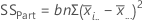TermDescription
bnumber of operators
nnumber of replicates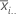mean for each part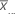grand mean
SSOperator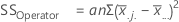TermDescription
anumber of parts
nnumber of replicates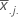mean for each operatorgrand mean
SSPart*Operator

SSPart*Operator = SSTotal – (SSPart + SSOperator + SSRepeatability)

SSRepeatability
When the interaction term is in the ANOVA table, use the following formula for the sum of squares for repeatability: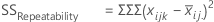When the interaction term is not in the ANOVA table, use the following formula for the sum of squares for repeatability: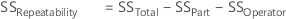TermDescription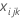each observation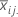mean of each factor level
SSTotal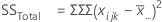TermDescriptioneach observationgrand mean

## Degrees of freedom (DF)

The degrees of freedom (DF) for each SS (sums of squares). In general, DF measures how much information is available to calculate each SS.

DFPart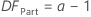TermDescription
anumber of parts
DFOperator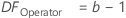TermDescription
bnumber of operators
DFPart*Operator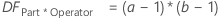TermDescription
anumber of parts
bnumber of operators
DFRepeatability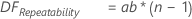TermDescription
anumber of parts
bnumber of operators
nnumber of replicates
DFTotal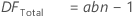TermDescription
anumber of parts
bnumber of operators
nnumber of replicates

## Mean squares (MS)

The mean squares (MS) is the variability in the data from different sources. MS accounts for the fact that different sources have different numbers of levels or possible values.

MSPart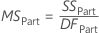MSOperator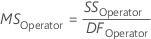MSPart*Operator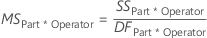MSRepeatability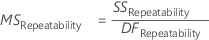## F

The F-statistic is used to determine whether the effects of Operator, Part, or Operator*Part are statistically significant.

FPart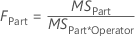FOperator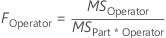FPart*Operator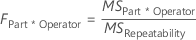## p-value

The p-value is the probability of obtaining a test statistic (such as the F-statistic) that is at least as extreme as the value that is calculated from the sample, if the null hypothesis is true.

By using this site you agree to the use of cookies for analytics and personalized content.  Read our policy